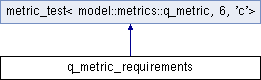q_metric_requirements Struct Reference

`#include <q_metrics_test.h>`

Inheritance diagram for q_metric_requirements:## Static Public Member Functions

static void create_expected (metric_set_t &metrics, const model::run::info &run_info)

static void create_summary (model::summary::run_summary &summary)Static Public Member Functions inherited from metric_test< model::metrics::q_metric, 6, 'c'>
static std::string name ()

static std::vector< T > to_vector (const T(&vals)[N])

static std::vector< T > to_vector (const sparse_value< T, Size >(&vals)[N])

## Additional Inherited MembersPublic Types inherited from metric_test< model::metrics::q_metric, 6, 'c'>
enum  Checks

typedef model::metrics::q_metric metric_t

typedef model::metric_base::metric_set< model::metrics::q_metricmetric_set_t

typedef metric_set_t::const_iterator const_iterator

## Detailed Description

This generator creates a contrived run metrics and q-metrics to test specific requirements

model::metrics::q_metric
Note
Version 6

## Member Function Documentation

 static void create_expected ( metric_set_t & metrics, const model::run::info & run_info )
inlinestatic

Create the expected metric set

Parameters
 metrics destination metric set run_info run info
 static void create_summary ( model::summary::run_summary & summary )
inlinestatic

Create the expected run_summary to test the Q-metrics requirements

Parameters
 summary run summary

The documentation for this struct was generated from the following file: